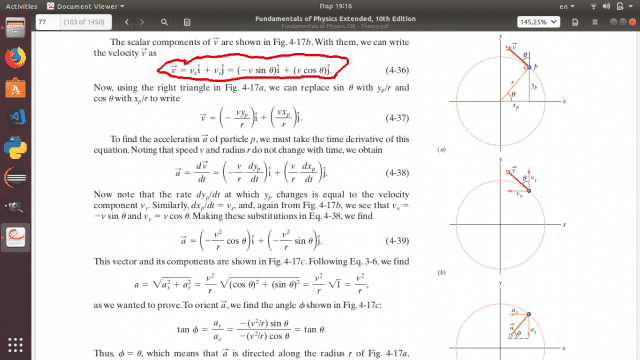# Uniform Circular Motion: some help with the math proof?

• babaliaris

#### babaliarisI can not understand why ##v_x = -|v|sin(θ)## and ##v_y = |v|cos(θ)##
I'm asking about the θ angle. If i move the vector v with my mind to the origin
i get that the angle between x'x and the vector in anti clock wise, it's 90+θ not just θ. So why is he using just θ? Does the minus in v_x somehow make the result the same when using just θ?

Also I thought that sin goes with the v_y and cos goes with v_x not the opposite...

#### Attachments

Also I thought that sin goes with the v_y and cos goes with v_x not the opposite...
If you are using the angle between v and the x-axis, that is right. So vx = |v|cos(90+θ) and vy = |v|sin(90+θ).
Do you see how that turns into -|v|sinθ and |v|cosθ?

•babaliaris
If you are using the angle between v and the x-axis, that is right. So vx = |v|cos(90+θ) and vy = |v|sin(90+θ).
Do you see how that turns into -|v|sinθ and |v|cosθ?

No I don't, this is what I want to understand. Can you show me mathematically how is this true? I need to see it written in math (this is important) else I can't understand why.

Do you know the formulae cos(A+B) = cosAcosB - sinAsinB
and sin(A+B) = sinAcosB + cosAsinB ?

•babaliaris
Do you know the formulae cos(A+B) = cosAcosB - sinAsinB
and sin(A+B) = sinAcosB + cosAsinB ?

Oh, I forgot about that... Now i can see why.

$$cos(90+θ) = cos(90)cos(θ) - sin(90)sin(θ) = 0 - sin(θ) \\ sin(90+θ) = sin(90)cos(θ) + cos(90)sin(θ) = cos(θ) + 0$$

Thank you! I was truly struggling finding this one!